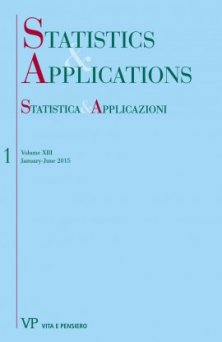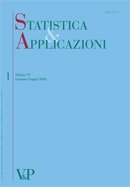Libri di T.T. Alodat - libri Statistica & Applicazioni

# T.T. Alodat

### Titoli dell'autore

A Generalized multivariate skew-normal distribution with applications to spatial and regression predictions digitalYear: 2015
In this paper, a generalization to the multivariate skew-normal distribution of Arnold and Beaver (2002) is proposed. Also several distributional properties of the proposed distribution are explored. The proposed distribution has been used to define a stochastic process called the generalized-skew Gaussian process...
Moving extreme ranked set sampling for simple linear regression
Free
digitalYear: 2009
The moving extreme ranked set sampling, introduced by Alodat and Al-Saleh (2001), is a modification of the well known ranked set sampling approach that was proposed by McIntyre (1952). In this paper, we suggest new estimators for the simple linear regression parameters under the moving extreme ranked set sampling scheme. Moreover, we show that the proposed estimators are more efficient than their counterparts using the simple random sampling approach. We illustrate our ideas and thoughts via simulation and data analysis and conduct a comparison between our approach and the traditional ones. Keywords: Moving ranked set sampling, Ranked set sampling, Simple linear regression.